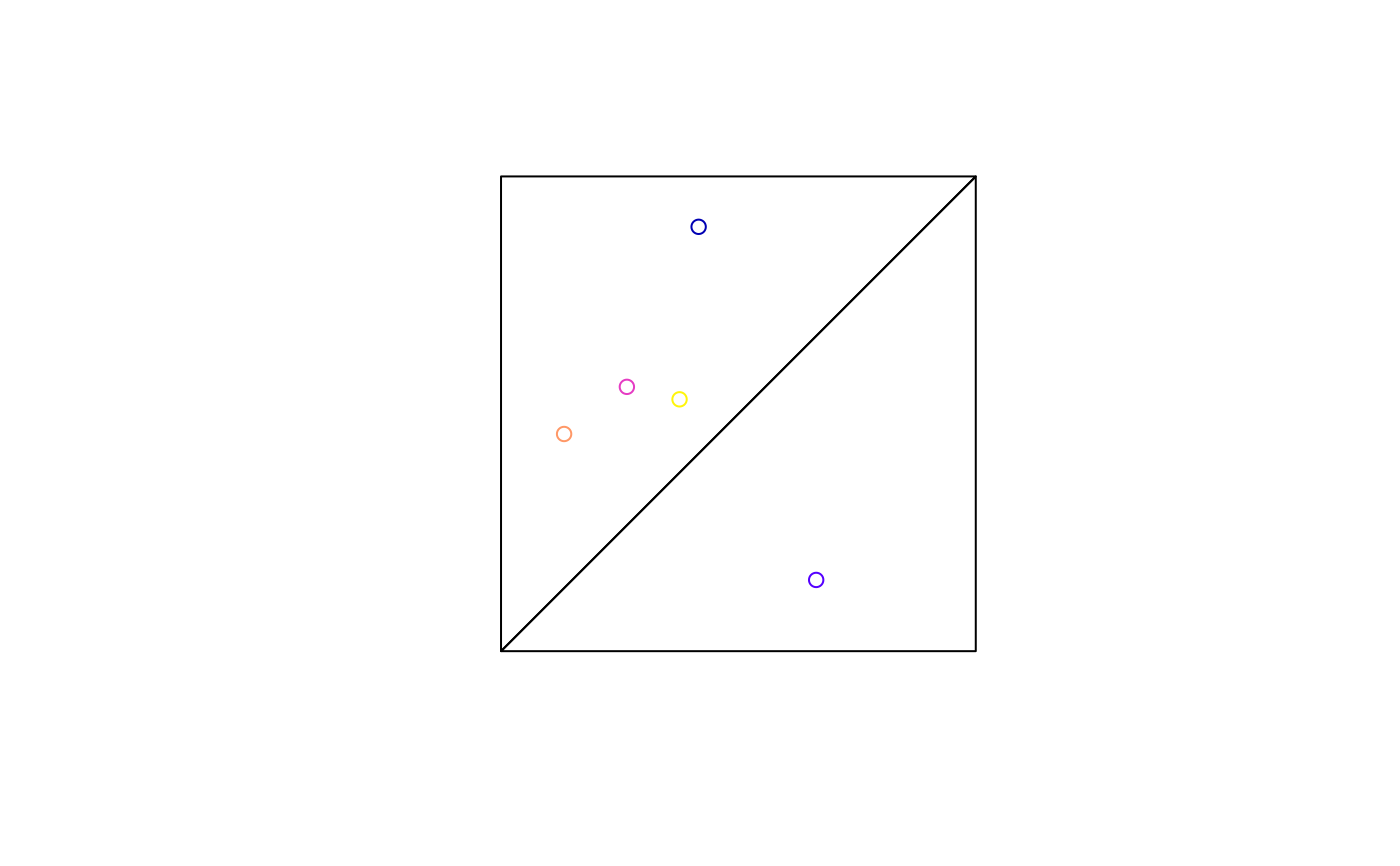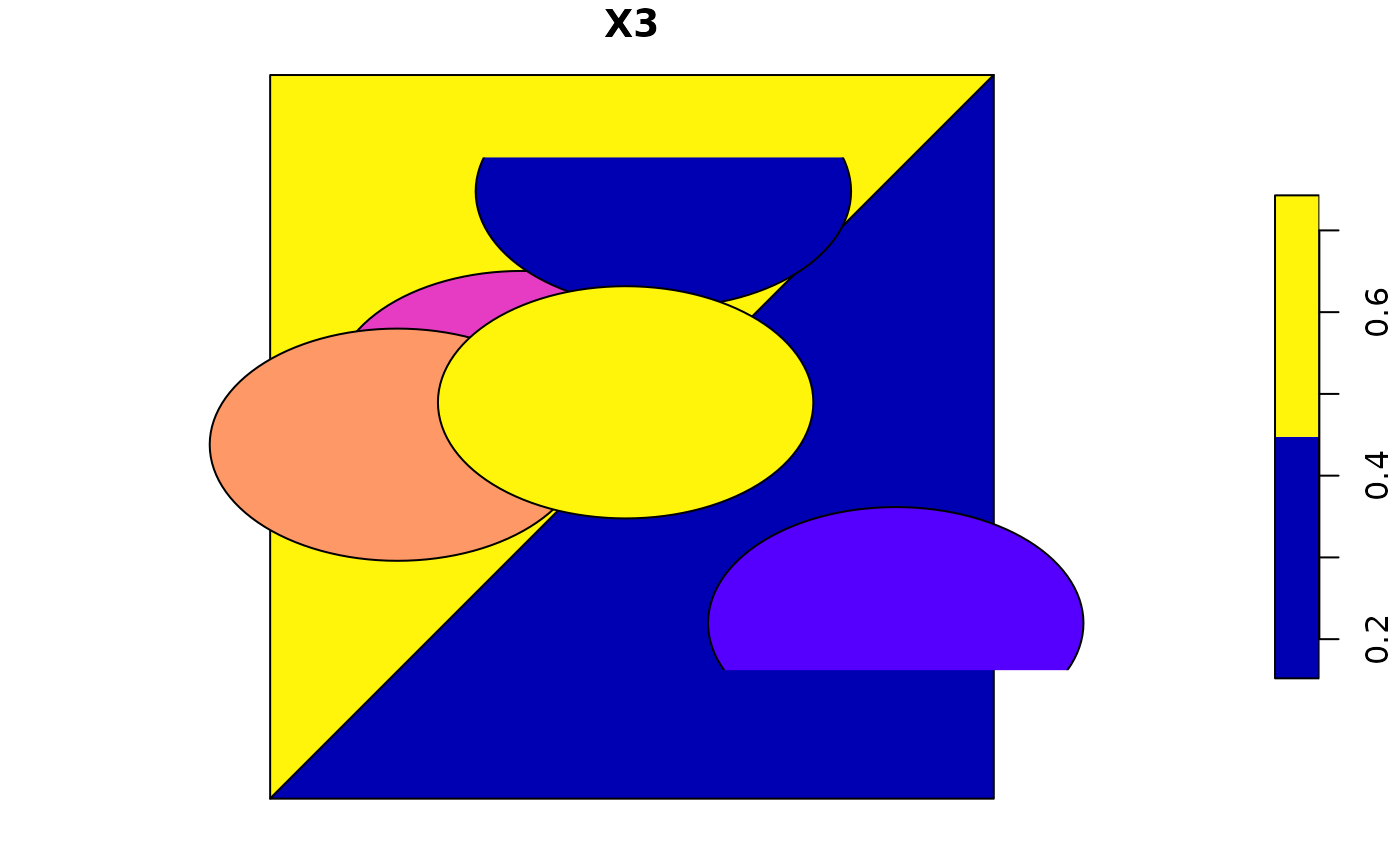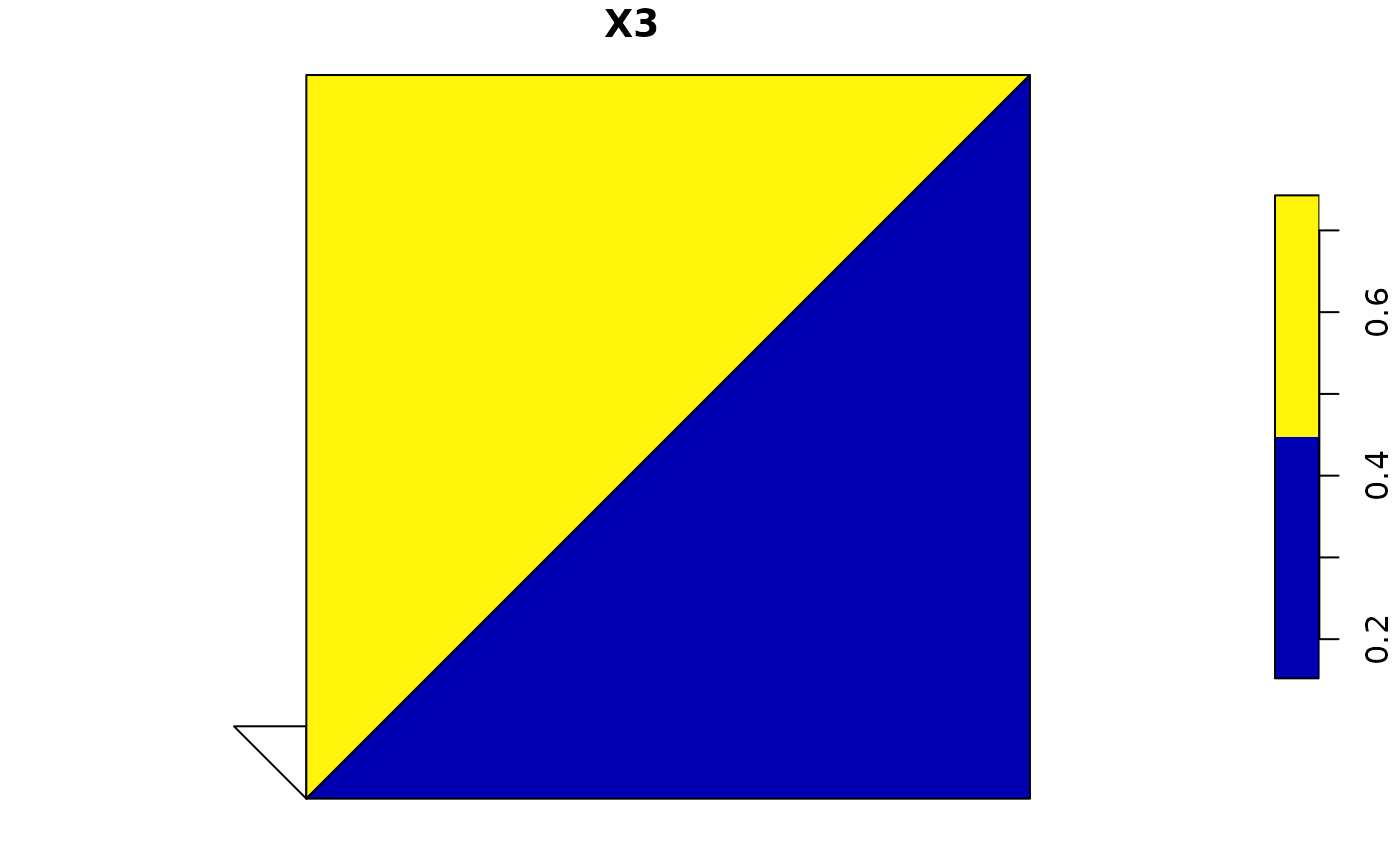aggregate an sf object, possibly union-ing geometries

# S3 method for sf
aggregate(
x,
by,
FUN,
...,
do_union = TRUE,
simplify = TRUE,
join = st_intersects
)

## Arguments

x

object of class sf

by

either a list of grouping vectors with length equal to nrow(x) (see aggregate), or an object of class sf or sfc with geometries that are used to generate groupings, using the binary predicate specified by the argument join

FUN

function passed on to aggregate, in case ids was specified and attributes need to be grouped

...

arguments passed on to FUN

do_union

logical; should grouped geometries be unioned using st_union? See details.

simplify

logical; see aggregate

join

logical spatial predicate function to use if by is a simple features object or geometry; see st_join

## Value

an sf object with aggregated attributes and geometries; additional grouping variables having the names of names(ids) or are named Group.i for ids[[i]]; see aggregate.

## Details

In case do_union is FALSE, aggregate will simply combine geometries using c.sfg. When polygons sharing a boundary are combined, this leads to geometries that are invalid; see https://github.com/r-spatial/sf/issues/681.

## Note

Does not work using the formula notation involving ~ defined in aggregate.

## Examples

m1 = cbind(c(0, 0, 1, 0), c(0, 1, 1, 0))
m2 = cbind(c(0, 1, 1, 0), c(0, 0, 1, 0))
pol = st_sfc(st_polygon(list(m1)), st_polygon(list(m2)))
set.seed(1985)
d = data.frame(matrix(runif(15), ncol = 3))
p = st_as_sf(x = d, coords = 1:2)
plot(pol)(p_ag1 = aggregate(p, pol, mean))
#> Simple feature collection with 2 features and 1 field
#> Geometry type: POLYGON
#> Dimension:     XY
#> Bounding box:  xmin: 0 ymin: 0 xmax: 1 ymax: 1
#> CRS:           NA
#>          X3                       geometry
#> 1 0.5951766 POLYGON ((0 0, 0 1, 1 1, 0 0))
#> 2 0.2997908 POLYGON ((0 0, 1 0, 1 1, 0 0))
plot(p_ag1) # geometry same as pol
# works when x overlaps multiple objects in 'by':
p_buff = st_buffer(p, 0.2)(p_ag2 = aggregate(p_buff, pol, mean)) # increased mean of second
#> Simple feature collection with 2 features and 1 field
#> Geometry type: POLYGON
#> Dimension:     XY
#> Bounding box:  xmin: 0 ymin: 0 xmax: 1 ymax: 1
#> CRS:           NA
#>          X3                       geometry
#> 1 0.5951766 POLYGON ((0 0, 0 1, 1 1, 0 0))
#> 2 0.5958297 POLYGON ((0 0, 1 0, 1 1, 0 0))
# with non-matching features
m3 = cbind(c(0, 0, -0.1, 0), c(0, 0.1, 0.1, 0))
pol = st_sfc(st_polygon(list(m3)), st_polygon(list(m1)), st_polygon(list(m2)))
(p_ag3 = aggregate(p, pol, mean))
#> Simple feature collection with 3 features and 1 field
#> Geometry type: POLYGON
#> Dimension:     XY
#> Bounding box:  xmin: -0.1 ymin: 0 xmax: 1 ymax: 1
#> CRS:           NA
#>          X3                       geometry
#> 1        NA POLYGON ((0 0, 0 0.1, -0.1 ...
#> 2 0.5951766 POLYGON ((0 0, 0 1, 1 1, 0 0))
#> 3 0.2997908 POLYGON ((0 0, 1 0, 1 1, 0 0))
plot(p_ag3)# In case we need to pass an argument to the join function:
(p_ag4 = aggregate(p, pol, mean,
join = function(x, y) st_is_within_distance(x, y, dist = 0.3)))
#> Simple feature collection with 3 features and 1 field
#> Geometry type: POLYGON
#> Dimension:     XY
#> Bounding box:  xmin: -0.1 ymin: 0 xmax: 1 ymax: 1
#> CRS:           NA
#>          X3                       geometry
#> 1        NA POLYGON ((0 0, 0 0.1, -0.1 ...
#> 2 0.5951766 POLYGON ((0 0, 0 1, 1 1, 0 0))
#> 3 0.5999887 POLYGON ((0 0, 1 0, 1 1, 0 0))